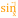# Arc Sine

 Block Group: Trigonometric Functions Icon:The Arc Sine block returns the arcsine, or inverse sine, of a number, in degrees. The arcsine of n is the angle for which n is the sine.

For information on using dataflow blocks, see Dataflow.

## Input/Output Property

The following property of the Arc Sine block can take input and give output.

• input (number)

input defines the sine to get the angle for. Must be between −1 and 1.

## Output Property

The following property of the Arc Sine block can give output but cannot take input.

• output (number)

output returns the arcsine of the input, in degrees.

## Example

The following image shows an example of the Arc Sine block. In this example, the sine is 1, and the arcsine is 90 degrees.